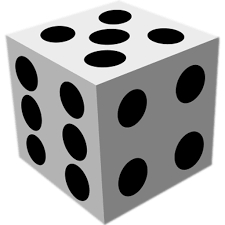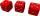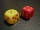# One dice

Calculate the probability of one dice roll with the numbers 1, 2, 3, 4, 5, 6 on the walls. Write the results in a notebook in the shape of a fraction in the basic form: 2/3. a, The number 1 falls on the cube. b, The number 5 falls on the cube. c, An even number falls on the cube. d, An odd number falls on the cube. e, A number divisible by three falls on the cube. f, The number divisible by two drops on the cube g, A number greater than 2 falls on the cube. h, A number less than 6 falls on the cube. i, A number greater than two and less than five will fall on the cube. j, An even number less than four drops on the cube.

a =  0.1667
b =  0.1667
c =  0.5
d =  0.5
e =  0.3333
f =  0.5
g =  0.6667
h =  0.8333
i =  0.3333
j =  0.1667

### Step-by-step explanation:

$a=\frac{1}{6}=\frac{1}{6}=0.1667$
$b=\frac{1}{6}=\frac{1}{6}=0.1667$Did you find an error or inaccuracy? Feel free to write us. Thank you!Tips to related online calculators
Need help to calculate sum, simplify or multiply fractions? Try our fraction calculator.
Would you like to compute count of combinations?

## Related math problems and questions:

• Air draftThe numbers 1,2,3,4,5 are written on five tickets on the table. Air draft randomly shuffled the tickets and composed a 5-digit number from them. What is the probability that he passed: and, the largest possible number b, the smallest possible number c, a
• Roll the diceWhat is the probability that if we roll the dice, a number less than 5 falls?
• Three diceWhat is the probability that when we roll three dice, we roll the numbers 1,2,3?
• Sum on diceWe have two dice. What is the greater likelihood of fall a total sum 7 or 8 ? (write 7, 8 or 0 if the probabilities are the same)?
• We rollWe roll two dice A. - what is the probability that the sum of the falling numbers is at most 4 B. - is at least 10 C. - is divisible by 5?
• Fall sum or sameFind the probability that if you roll two dice, it will fall the sum of 10, or the same number will fall on both dice.
• Chocolate rollThe cube of 5 cm chocolate roll weighs 30 g. How many calories will contain the same chocolate roller of a prism shape with a length of 0.5 m whose cross-section is an isosceles trapezoid with bases 25 and 13 cm and legs 10 cm? You know that 100 g of this
• Sum of fall dicesWhat is the probability that the sum of 9 will fall on a roll of two dice? Hint: write down all the pairs that can occur as follows: 11 12 13 14 15. . 21 22 23 24. .. . 31 32. .. . . . . . .. . 66, count them, it's the variable n variable m: 36, 63,. .. .
• Two diceWe roll two dice. What is the probability that the sum of the falling numbers is greater than 3?
• DicesWe will throw two dice. What is the probability that the ratio between numbers on first and second dice will be 1:2?
• Fractions and mixed numerals(a) Convert the following mixed numbers to improper fractions. i. 3 5/8 ii. 7 7/6 (b) Convert the following improper fraction to a mixed number. i. 13/4 ii. 78/5 (c) Simplify these fractions to their lowest terms. i. 36/42 ii. 27/45 2. evaluate the follow
• Six on diceWhat is the probability that when throwing two dice will fall at least one six?
• Divisible by 5How many three-digit odd numbers divisible by 5, which are in place ten's number 3?
• Equation with fractionsWhat is the simplified form of the equation fraction 4 over 5 n minus fraction 1 over 5 equals fraction 2 over 5 n? n = -2 n = 4 n = fraction 1 over 2
• One threeWe throw two dice. What is the probability that max one three falls?
• Three digits numberFrom the numbers 1, 2, 3, 4, 5 create three-digit numbers that digits not repeat and number is divisible by 2. How many numbers are there?
• PIN codePIN on Michael credit card is a four-digit number. Michael told this to his friend: • It is a prime number - that is, a number greater than 1, which is only divisible by number one and by itself. • The first digit is larger than the second. • The second d